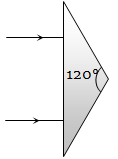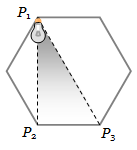An isosceles prism of angle 120° has a refractive index of 1.44. Two parallel monochromatic rays enter the prism parallel to each other in air as shown. The rays emerging from the opposite faces :(a) Are parallel to each other

(b) Are diverging

(c) Make an angle $2{\mathrm{sin}}^{-1}\left(0.72\right)$ with each other

(d) Make an angle $2\left\{{\mathrm{sin}}^{-1}\left(0.72\right)-30°\right\}$ with each other

Concept Questions :-

Prisms
High Yielding Test Series + Question Bank - NEET 2020

Difficulty Level:

A ray of light is incident on the hypotenuse of a right-angled prism after travelling parallel to the base inside the prism. If $\mu$ is the refractive index of the material of the prism, the maximum value of the base angle for which light is totally reflected from the hypotenuse is

(a) ${\mathrm{sin}}^{-1}\left(\frac{1}{\mu }\right)$                             (b) ${\mathrm{tan}}^{-1}\left(\frac{1}{\mu }\right)$

(c) ${\mathrm{sin}}^{-1}\left(\frac{\mu -1}{\mu }\right)$                         (d) ${\mathrm{cos}}^{-1}\left(\frac{1}{\mu }\right)$

Concept Questions :-

Total internal reflection
High Yielding Test Series + Question Bank - NEET 2020

Difficulty Level:

A plano-convex lens when silvered in the plane side behaves like a concave mirror of focal length 30 cm. However, when silvered on the convex side it behaves like a concave mirror of focal length 10 cm. Then the refractive index of its material will be

(a) 3.0                                (b) 2.0

(c) 2.5                                (d) 1.5

Concept Questions :-

Lenses
High Yielding Test Series + Question Bank - NEET 2020

Difficulty Level:

A ray of light travels from an optically denser to rarer medium. The critical angle for the two media is C. The maximum possible deviation of the ray will be

(a) $\left(\frac{\mathrm{\pi }}{2}-C\right)$                          (b) 2C

(c) $\mathrm{\pi }-2\mathrm{C}$                             (d) $\mathrm{\pi }-\mathrm{C}$

Concept Questions :-

Total internal reflection
High Yielding Test Series + Question Bank - NEET 2020

Difficulty Level:

An astronaut is looking down on earth's surface from a space shuttle at an altitude of . Assuming that the astronaut's pupil diameter is 5 mm and the wavelength of visible light is 500 nm. The astronaut will be able to resolve linear object of the size of about

(a) 0.5 m                    (b) 5 m

(c) 50 m                      (d) 500 m

Concept Questions :-

Resolution of Optical devices
High Yielding Test Series + Question Bank - NEET 2020

Difficulty Level:

The average distance between the earth and moon is $38.6×{10}^{4}$ km. The minimum separation between the two points on the surface of the moon that can be resolved by a telescope whose objective lens has a diameter of 5 m with $\lambda =6000\stackrel{\circ }{Α}$ is

(a) 5.65 m                     (b) 28.25 m

(c) 11.30 m                    (d) 56.51 m

Concept Questions :-

Resolution of Optical devices
High Yielding Test Series + Question Bank - NEET 2020

Difficulty Level:

The distance of the moon from earth is . The eye is most sensitive to light of wavelength 5500 Å. The minimum separation between two points on the moon that can be resolved by a 500 cm telescope will be

(a) 51 m                         (b) 60 m

(c) 70 m                          (d) All the above

Concept Questions :-

Resolution of Optical devices
High Yielding Test Series + Question Bank - NEET 2020

Difficulty Level:

A light source is located at ${P}_{1}$ as shown in the figure. All sides of the polygon are equal. The intensity of illumination at ${{P}_{2}}_{}$is ${I}_{0}$ . What will be the intensity of illumination at ${P}_{3}$ ?(a) $\frac{3}{4}{I}_{0}$                                    (b) $\frac{{I}_{0}}{8}$

(c) $\frac{3}{8}{I}_{0}$                                        (d) $\frac{\sqrt{3}}{8}{I}_{0}$

Concept Questions :-

Reflection at spherical surface
High Yielding Test Series + Question Bank - NEET 2020

Difficulty Level:

A point object is moving on the principal axis of a concave mirror of focal length 24 cm towards the mirror. When it is at a distance of 60 cm from the mirror, its velocity is 9cm/sec. What is the velocity of the image at that instant

(a) 5cm/sec towards the mirror

(b) 4cm/sec towards the mirror

(c) 4cm/sec away from the mirror

(d) 9cm/sec away from the mirror

Concept Questions :-

Reflection at spherical surface
High Yielding Test Series + Question Bank - NEET 2020

Difficulty Level:

A concave mirror is placed on a horizontal table with its axis directed vertically upwards. Let O be the pole of the mirror and C is its centre of curvature. A point object is placed at C. It has a real image, also located at C. If the mirror is now filled with water, the image will be :

(a) Real, and will remain at C

(b) Real, and located at a point between C and $\infty$

(c) Virtual and located at a point between C and O

(d) Real, and located at a point between C and O

Concept Questions :-

Reflection at spherical surface